• Save

# Pie chart questions and answers for bank po

Last year 14.2K Views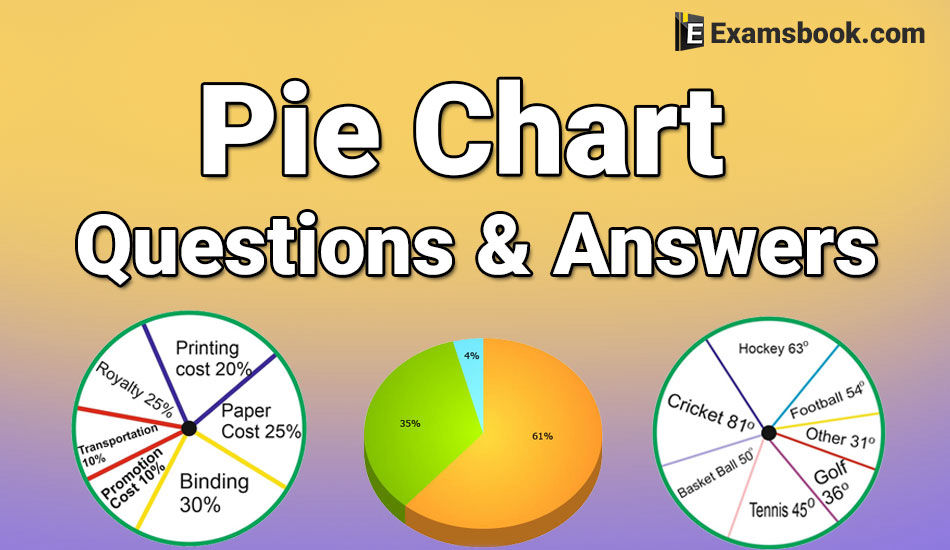Practice with pie chart questions and answers and build up your confidence. Here in this post, you can get important pie chart questions and answers for SSC, bank exams and competitive exams. Pie chart questions and answers are for those students who appeared in competitive exams.

Let's try to solve pie chart questions and answers yourself and track your results in this topic. These selective pie chart questions and answers are helpful to score better in SSC and Bank exams.

To use various types of formulas, you can solve pie chart questions. Here you can learn the way to use pie chart formula and examples with explanation and also practice line graph questions with answers.

## Pie Chart Questions and Answers for Competitive Exams

Direction (Questions 1 to 5): The circle-graph given here shows the spending of a country on various sports during a particular year. Study the graph carefully and answer the question given below it.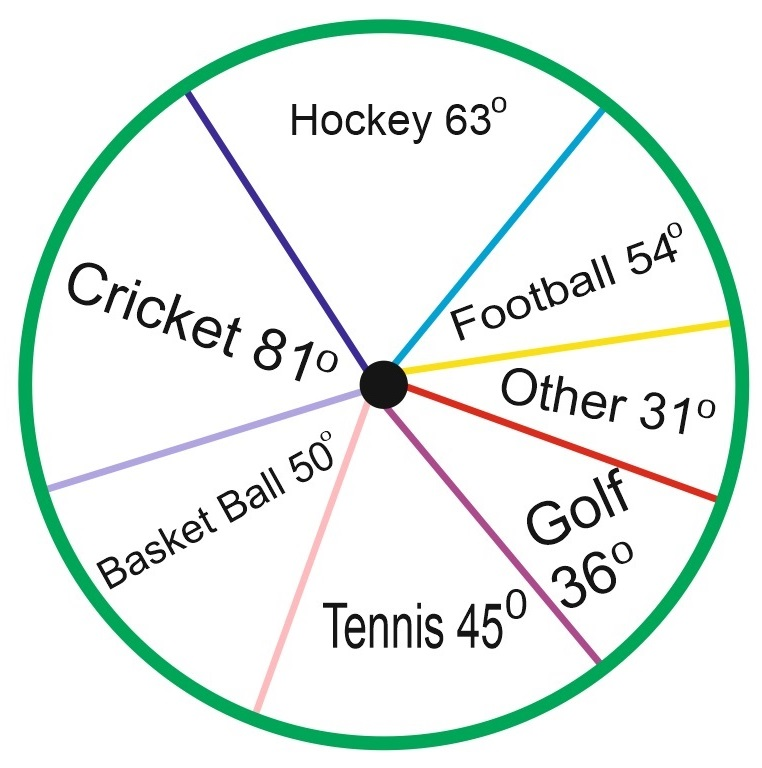Q.1. What percent of the total spending is spent on Tennis?

(A)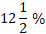(B)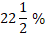(C) 25 %

(D) 45 %

Ans .   A

Q.2. How much percent is more spent on Hockey than that on Golf?

(A) 27 %

(B) 35 %

(C) 37.5 %

(D) 75 %

Ans .   D

Q.3. How much percent is less spent on Football than that on Cricket?

(A)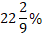(B) 27%

(C)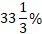(D) 30%

Ans .   C

Q.4. If the total amount spent on sports during the year was Rs. 2 crores, the amount spent on cricket and Hockey together was:

(A) Rs. 8,00,000

(B) Rs. 80,00,000

(C) Rs. 1,20,00,000

(D) Rs. 1,60,00,000

Ans .   B

Q.5. If the total amount spent on sports during the year be Rs. 1,80,00,000 the amount spent on Basketball exceeds that on Tennis by:

(A) Rs. 2,50,000

(B) Rs. 3,60,000

(C) Rs. 3,75,000

(D) Rs. 4,10,000

Ans .   A

Direction (6 to 10): Study the following pie-diagrams carefully and answer the question given below it.

PERCENTAGE COMPOSITION OF HUMAN BODY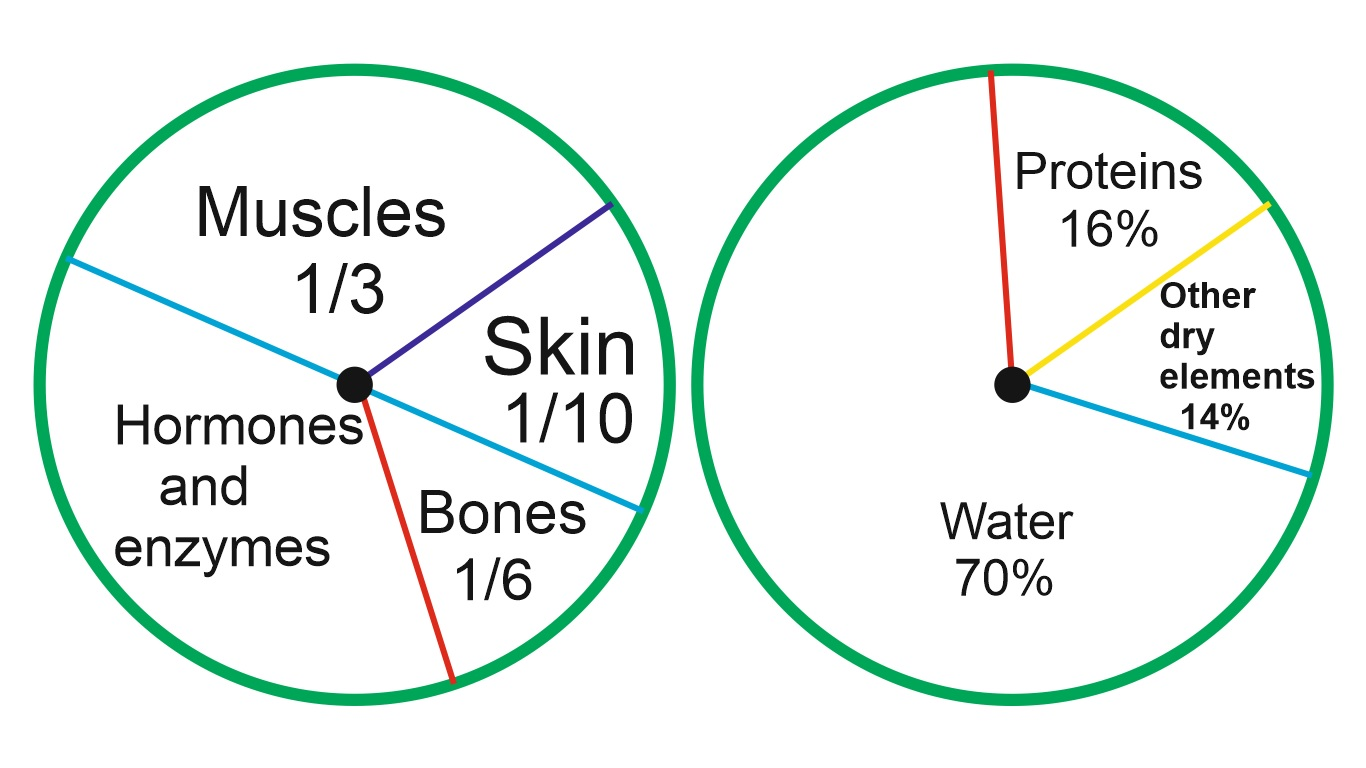Q.6. In the human body, what part is made of neither bones nor skin?

(A)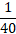(B)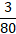(C)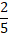(D) None of these

Ans .   D

Q.7. What is the ratio of the distribution of proteins in the muscles to that of the distribution of proteins in the bones?

(A) 1: 18

(B) 1: 2

(C) 2: 1

(D) 18: 1

Ans .   C

Q.8.what will be the quantity of water in the body of a person weighing 50 kg?

(A) 20 kg

(B) 35 kg

(C) 41 kg

(D) 42.5 kg

Ans .   B

Students can ask in comment section anything about pie chart questions and answers if they face any difficulty in pie chart questions and answers. Go to next page for more practice pie chart questions and answers for SSC and Bank Exams.